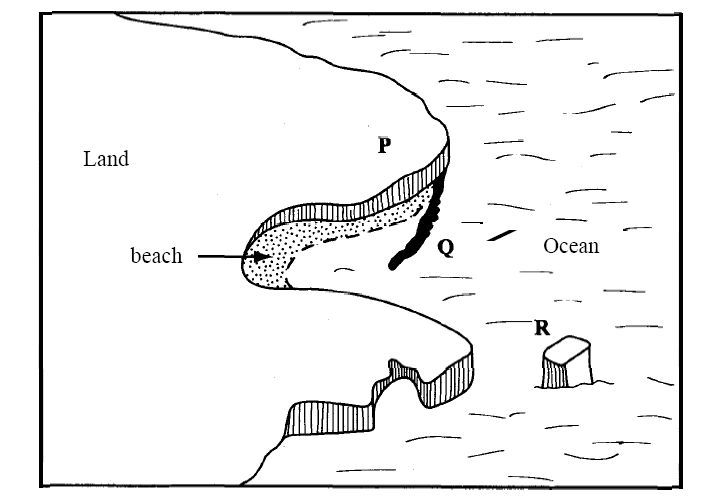# KCSE Geography Paper 1 2010

Find KCSE Geography Paper 1 2010. Kenya Certificate of Secondary Education Geography Paper 1 Past paper

SECTION A

Answer ALL the Questions in this Section

1. Give three components of the solar system.   (3 marks)

2.(a) Identify two types of high level clouds. .   (2 marks)

(b) Draw a well labelled diagram of a hydrological cycle. .   (5 marks)

3.(a) Give three causes of earthquakes. .   (3 marks)

(b) Name two major earthquake zones of the world. .   (3 marks)

4. (a) What is a rock? .   (2 marks)

(b) Give three characteristics of sedimentary rocks. .   (2 marks)

5.(a) The diagram below shows some coastal features.Name the features marked P, Q and R.    (3 marks)

(b) State two conditions necessary for the formation of a beach.   (2 marks)

SECTION B

Answer question 6 and any other TWO questions from this section.

6. Study the map of Homa Bay (1 50,000) sheet 12912 provided and answer the following questions.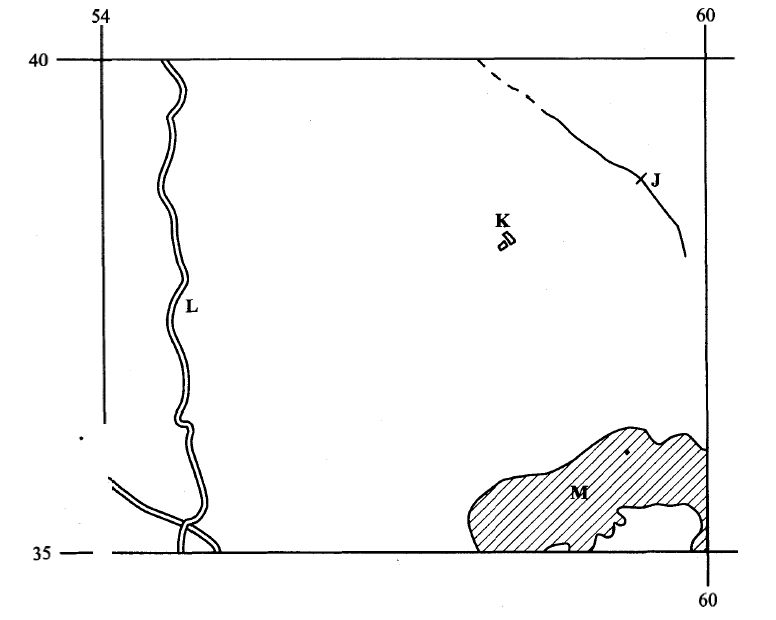(a) A pipeline is to be laid from Lake Victoria along the line marked X-Y.

(i) What is the length of the piping to be used? (Give your answer to the nearest 100 metres)          (2 marks)

(ii) Calculate the bearing of point Y from point X.      (2 marks)

(iii) Calculate the area of the part of Lake Victoria shown on the map excluding the marshy sections. (Give your answer in square kilometres).      (2 marks)

(b)  The rectangle below represents the area in the map extract bounded by Eastings 54 and 60 and Northings 35 and 40. Identify and name the features marked J, K, L and M.       (4 marks)

(c) (i) Explain three factors which have influenced the distribution of settlements in the area covered by the map.      (6 marks)

(ii) Citing evidence from the map, give two agricultural activities carried out in the area covered by the map.       (4 marks)

(d) Describe the drainage of the area covered by the map.       (5 marks)

7. The map below shows some vegetation regions of the world. Use it to answer questions (a) and (b). coniferous forest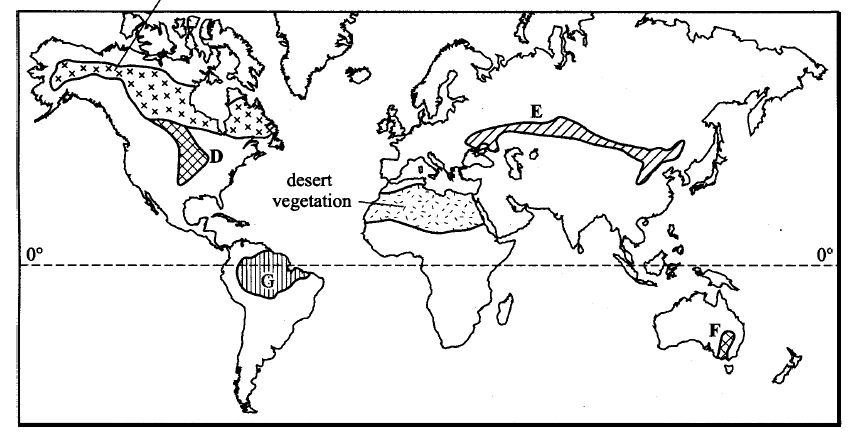(a) (i) Name the temperate grasslands marked D, E and F.    (3 marks)

(ii) Describe the characteristics of the natural vegetation found in the shaded area marked G.     (6 marks)

(b) Explain how climate has influenced the existence of the following types of vegetation shown on the map.

(i) Desert vegetation;      (4 marks)

(ii) Coniferous forest.     (4 marks)

(c) You are required to carry out a field study of the natural vegetation within your local environment.

(i) Apart from identifying the different types of plants, state three other activities you would carry out during the field study.      (3 marks)

(ii) How would you identify the different types of plants?     (3 marks)

(iii) State two ways in which the information collected during the field study would be useful to the local community. (2 marks)

8(a) Describe plucking as a process in glacial erosion.      (4 marks)

(b) Explain three conditions that lead to glacial deposition.      (6 marks)

(c) The diagram below shows features resulting from glacial deposition on a lowland area.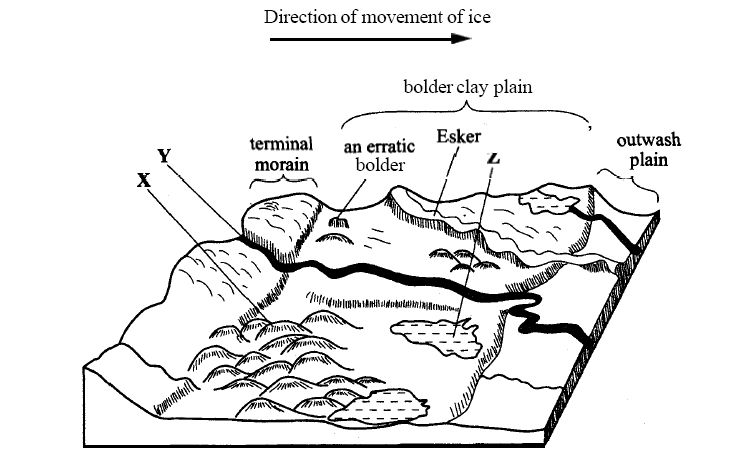(i) Name the features marked X, Y and Z.    (3 marks)

(ii) Describe how terminal moraine is formed.     (4 marks)

(d) Explain four positive effects of glaciation in lowland areas.    (8 marks)

9(a) Differentiate between river rejuvenation and river capture.      (2 marks)

(b) Give three features resulting from:

(i) river rejuvenation;    (3 marks)

(ii) river capture.           (3 marks)

(c) Explain the four ways through which a river transports its load.    (8 marks)

(d) You are planning to carry out a field study on the lower course of a river.

(i) Give three reasons why you would require a route map.     (3 marks)

(ii) State three characteristics of a river at the old stage that you are likely to observe during the field study.    (3 marks)

(iii) Give three follow-up activities you would be involved in after the field study.    (3 marks)

10. The diagram below represents a well developed soil profile. Use it to answer question (a).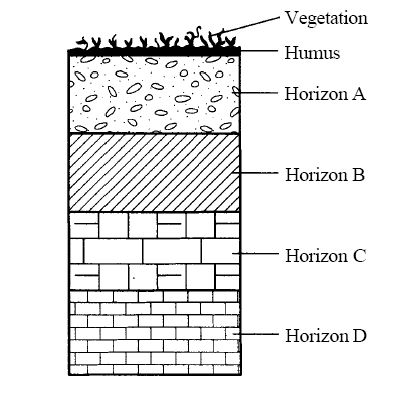(a) (i) Describe the characteristics of horizon B.     (3 marks)

(ii) Apart from humus, name three other components of soil.    (3 marks)

(iii) State three ways in which humus contributes to the quality of soil.    (3 marks)

(b) (i) Differentiate between soil structure and soil texture.     (2 marks)

(ii) Explain how the following factors influence the formation of soil:

<> topography;      (6 marks)

<> time.                  (2 marks)

(c) Explain how the following farming practices may lead to loss of soil fertility:

(i) overgrazing;                  (2 marks)

(ii) frequent ploughing;      (2 marks)

(iii) continuous irrigation.   (2 marks)